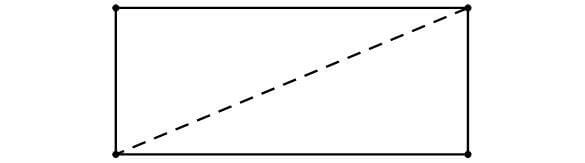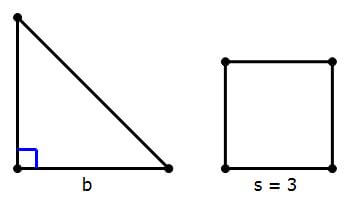# Area of a Triangle

As you may remember from high school, A=\dfrac{1}{2}bh, where b is the base and h is the height. If you are having trouble remembering this, simply remember that a rectangle has an area of A=bh, and that a triangle is half a rectangle.## Practice Question: Using the Area FormulaQ1. The figure on the left is an isosceles right triangle, and the figure on the right is a square of length 3. Find the value of b.

Statement #1: b is the length of the diagonal of the square.

Statement #2: the triangle and the square have the same area.

(A) Statement 1 alone is sufficient but statement 2 alone is not sufficient to answer the question asked.

(B) Statement 2 alone is sufficient but statement 1 alone is not sufficient to answer the question asked.

(C) Both statements 1 and 2 together are sufficient to answer the question but neither statement is sufficient alone.

(D) Each statement alone is sufficient to answer the question.